Question

Antenna Theory

(a) A 50 m long lossless transmission line with Zo = 50 ohms operating at 50 MHz is terminated with a load ZL = 90 + j 30 ohms. If the wave velocity is u =0.8 c,where cis the speed of light in vacuum, find:

ii. The input impedance

i. The reflection coefficient

(b) The Direct Broadcast System (DBS) satellite (at a distance of 39000 km)transmits at 13 GHz in the Ku Band, with a transmit power of 200 W, with a transmit antenna gain of 35 dB and with an IF (Intermediate Frequency)bandwidth of 30 MHz. The ground receiving station dish has a gain of 32 d Band sees an average background brightness temperature of Tb = 50 K. The receiver LNB (Low Noise Block) has a noise figure of 1.8 dB. Find:

i.The EIRP (Effective Isotropic Radiated Power)

ii.The power received at the input of the LNB

iii.The signal to Noise ratio at the LNB output

iv.Comment on what would happen if both transmit and receive antennas are replaced by typical monopole antennas.

(c) A certain two-port network is measured and the following scattering matrix is obtained:

[S]=\left[\begin{array}{cc} 0.3 \angle 0^{\circ} & 0.7 \angle 90^{\circ} \\ 0.7 \angle 90^{\circ} & 0.3 \angle 0^{\circ} \end{array}\right]

From the data determine:

i.If the network is reciprocal

ii.If the network is lossless

(d) Briefly describe how the following RF systems operate:

i.Wilkinson power divider

ii.CirculatorVerified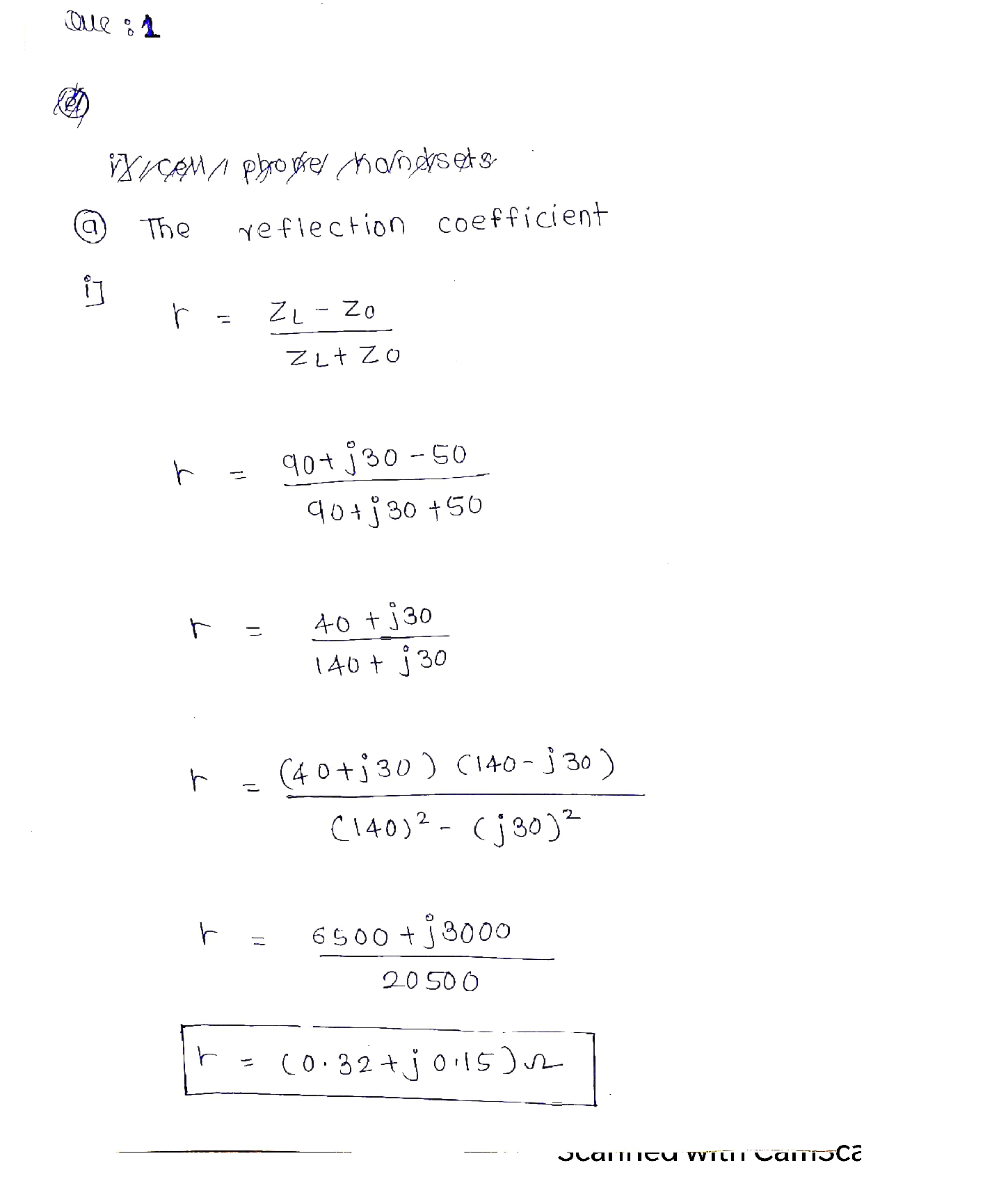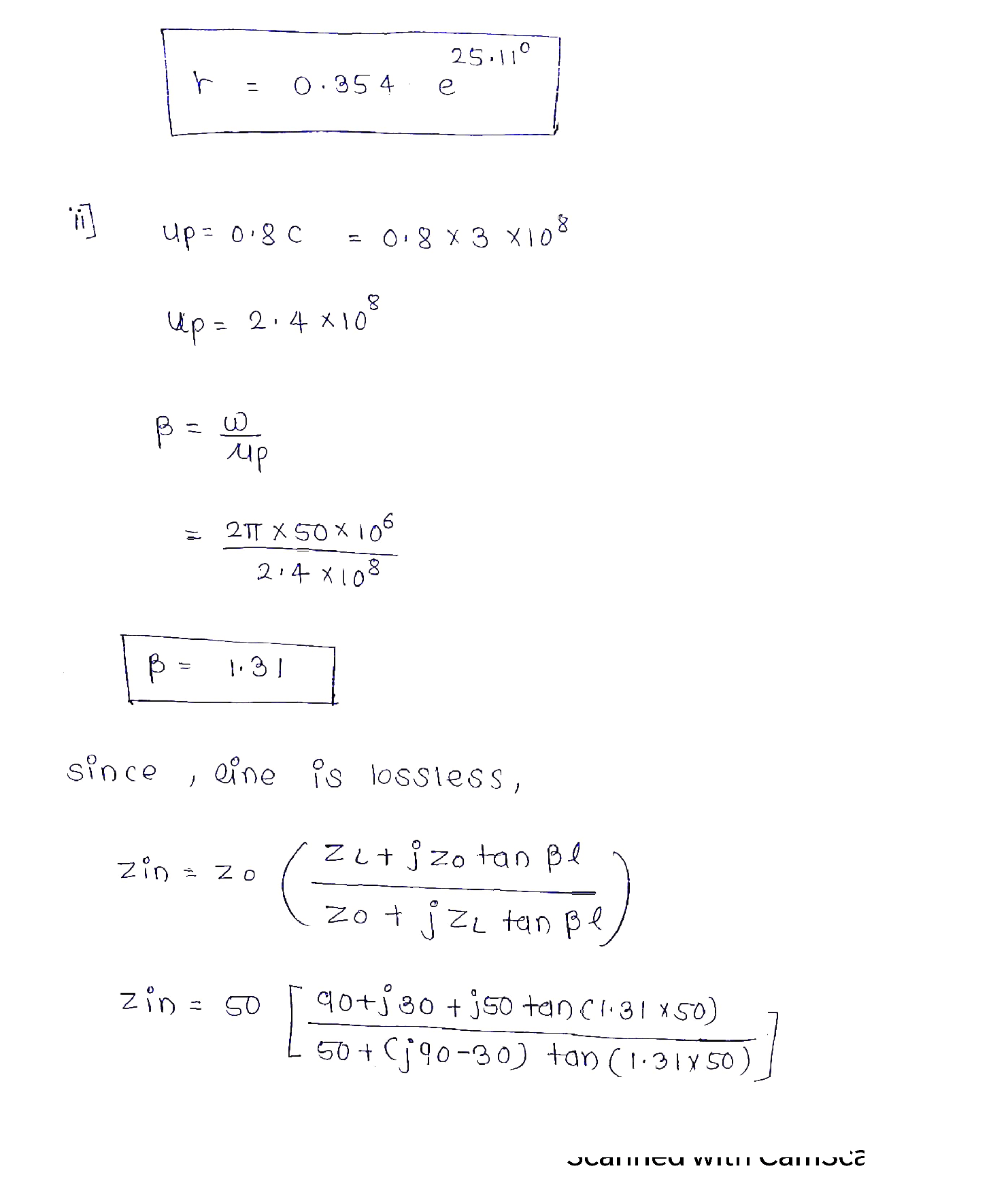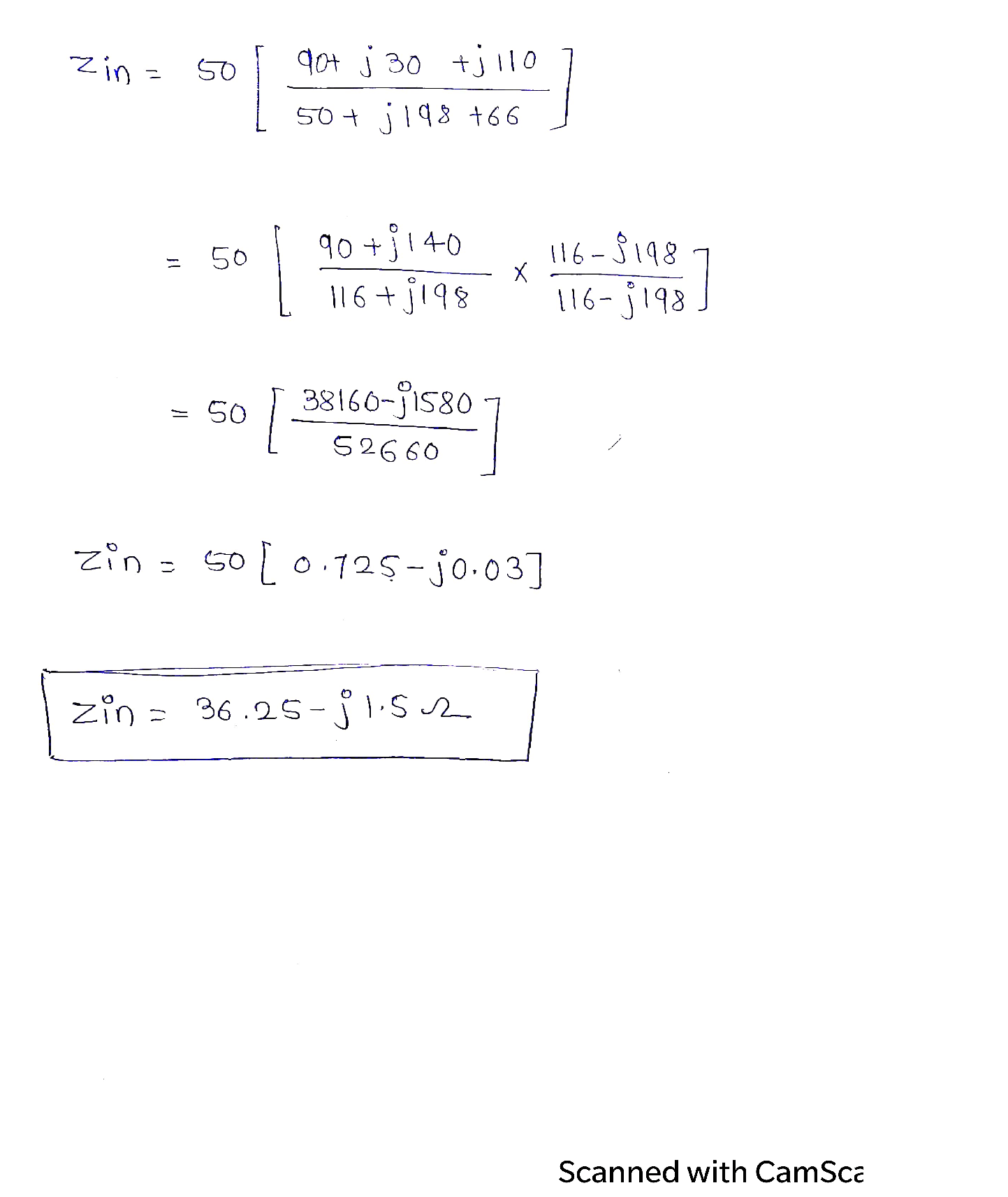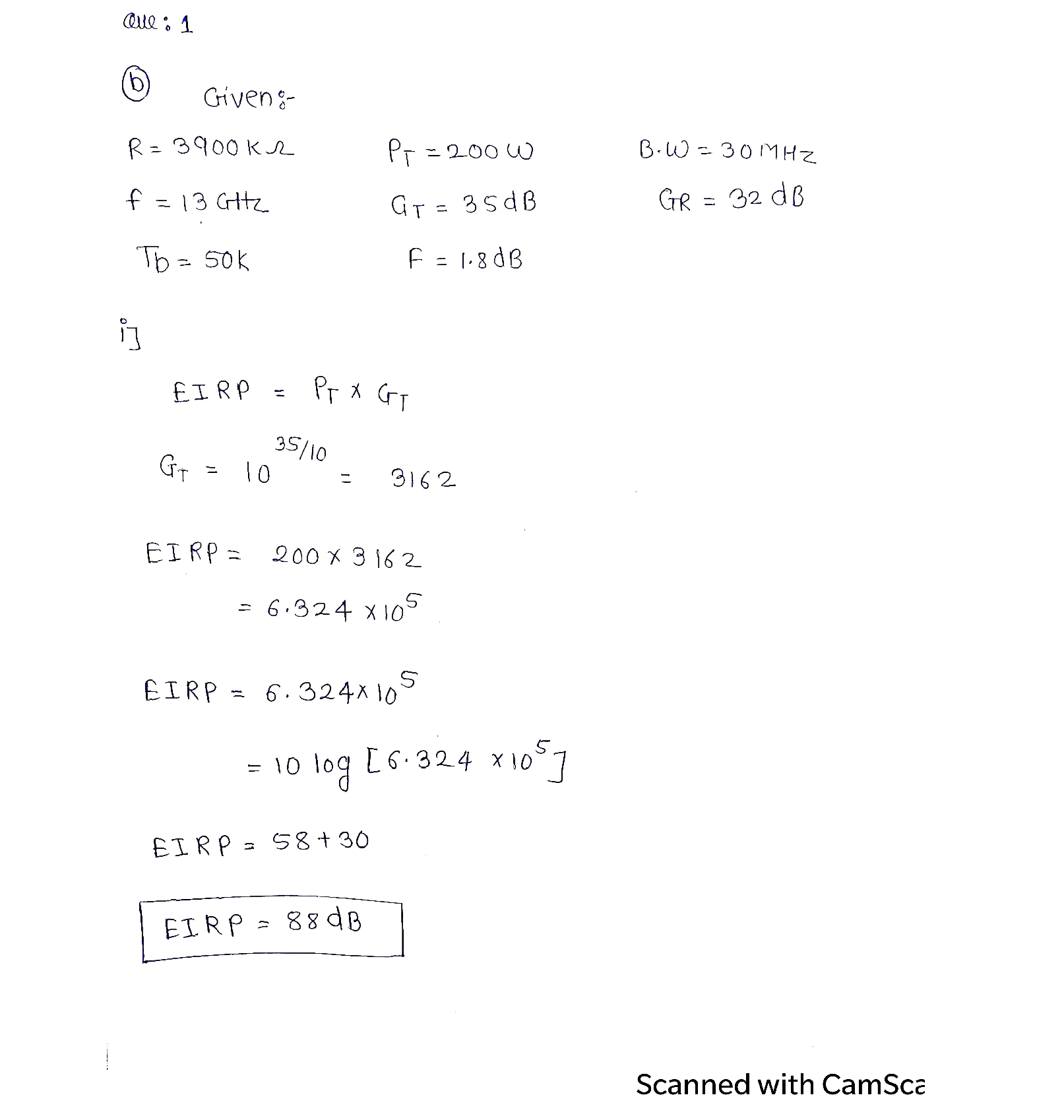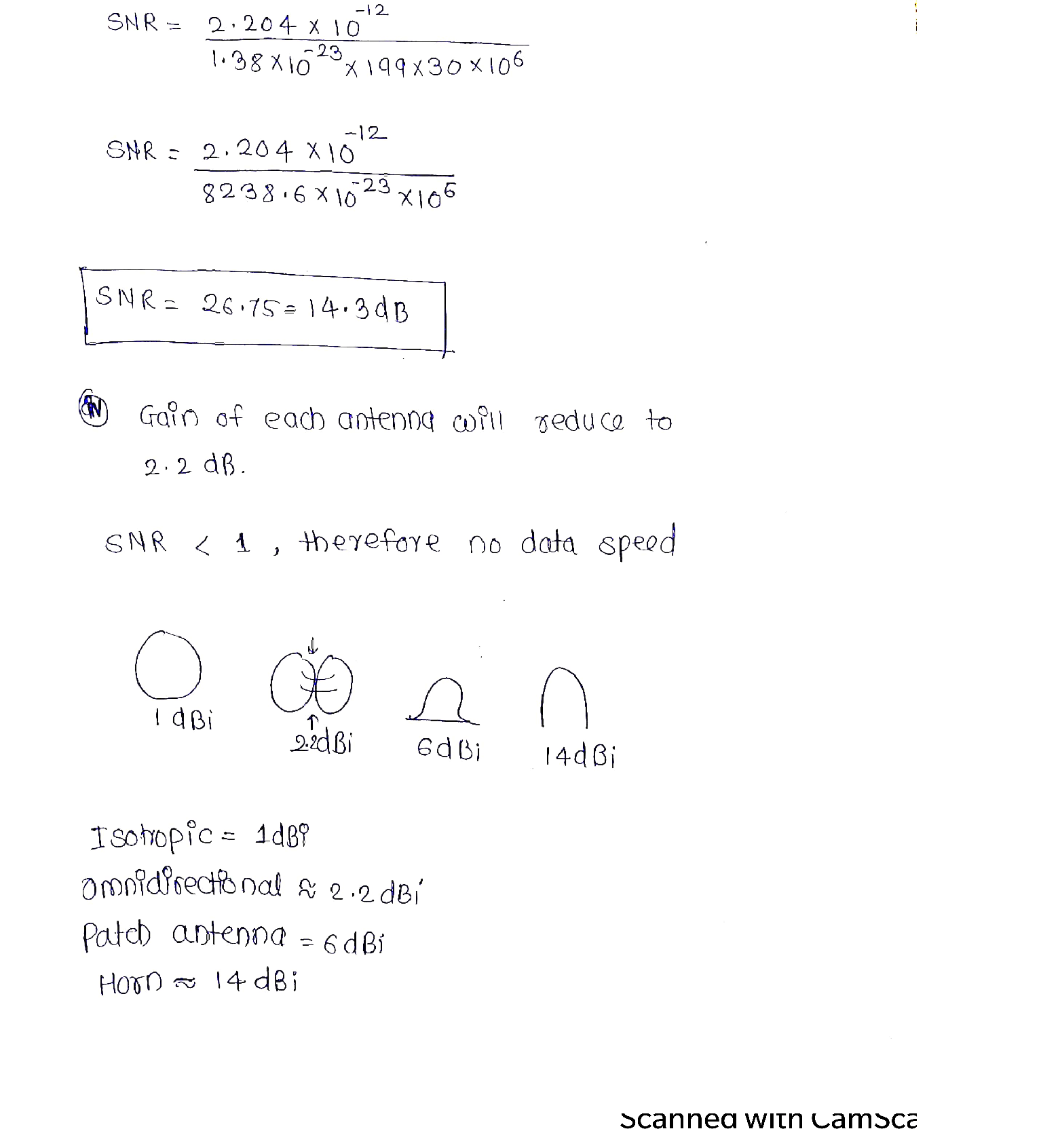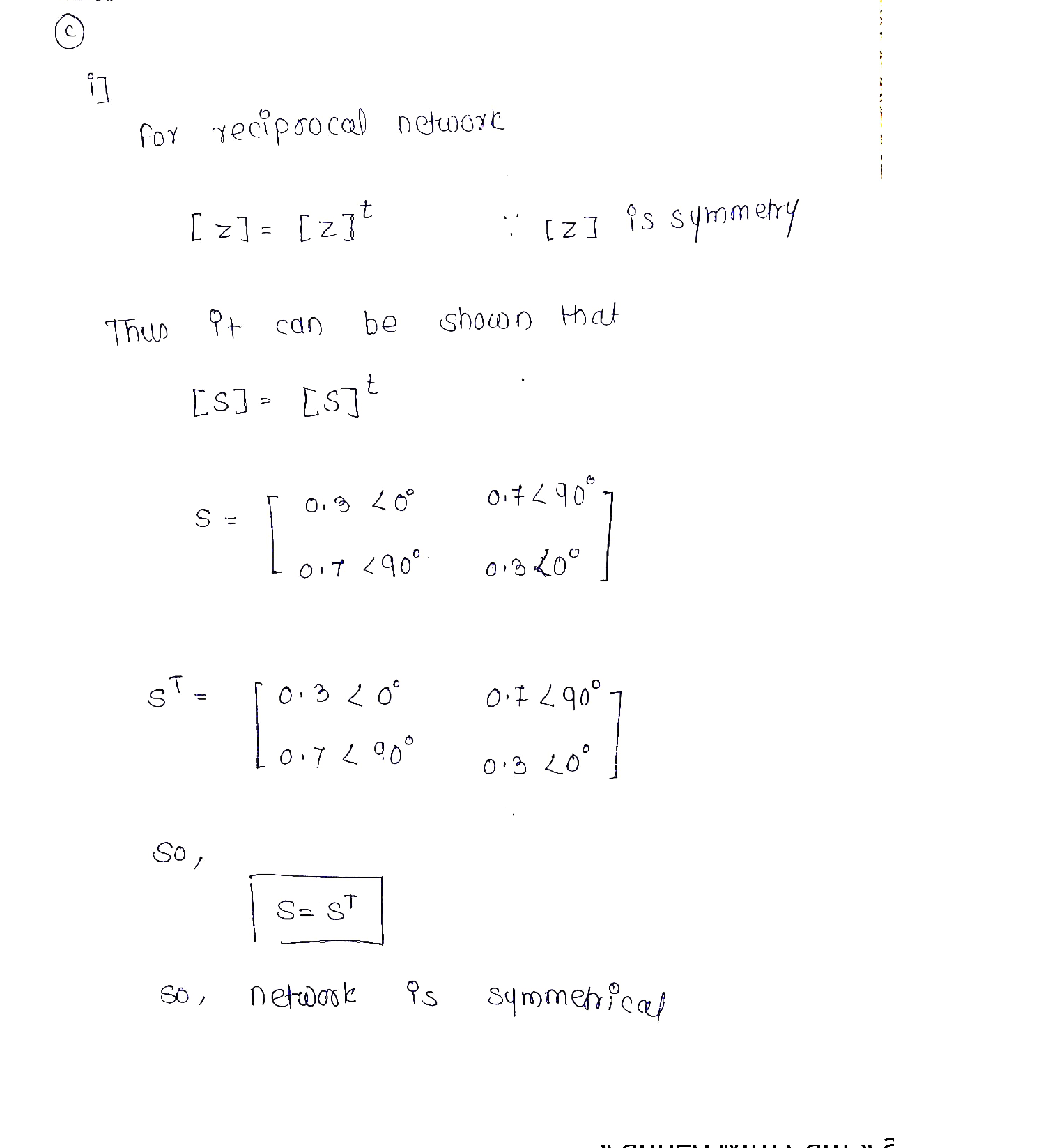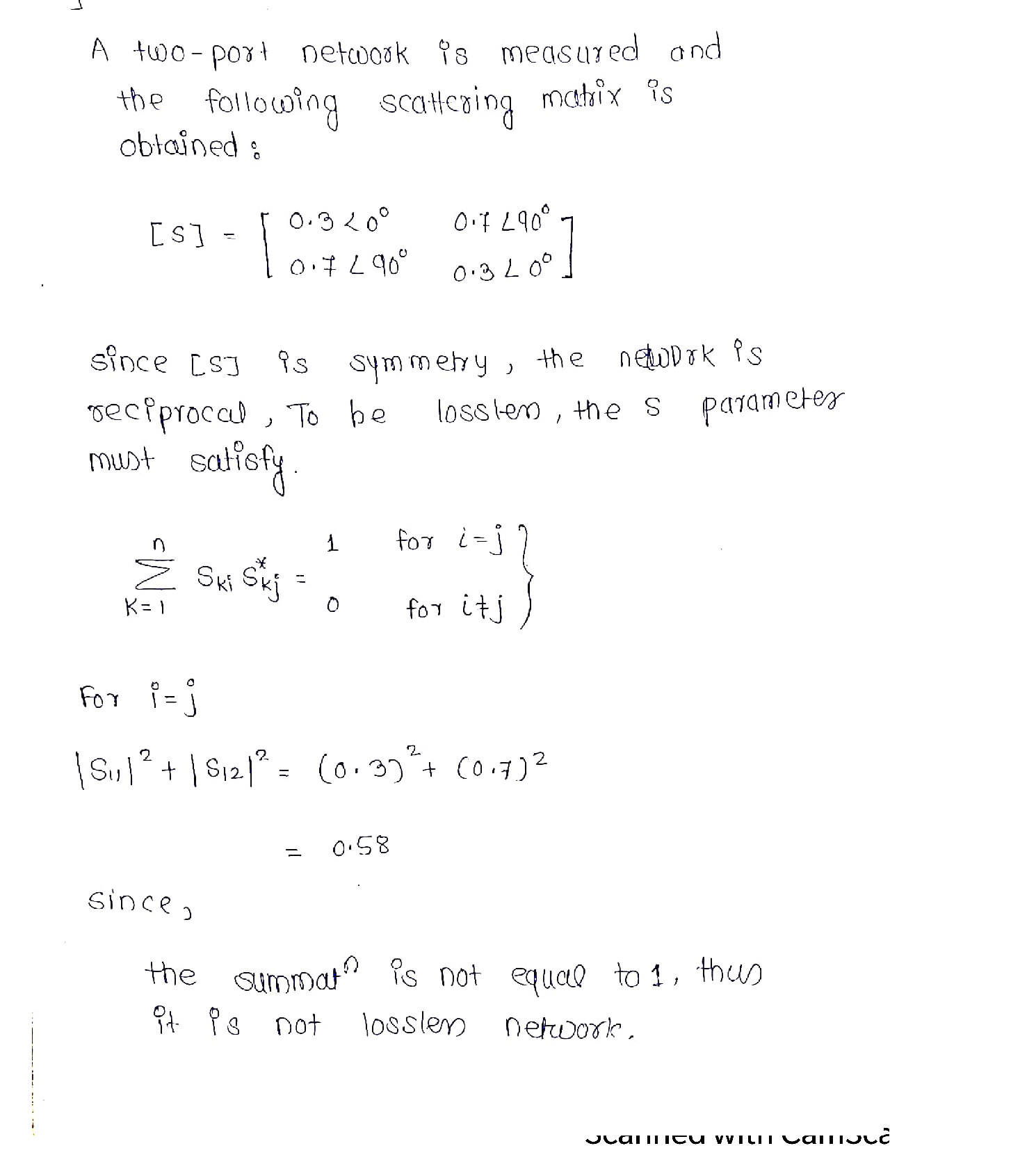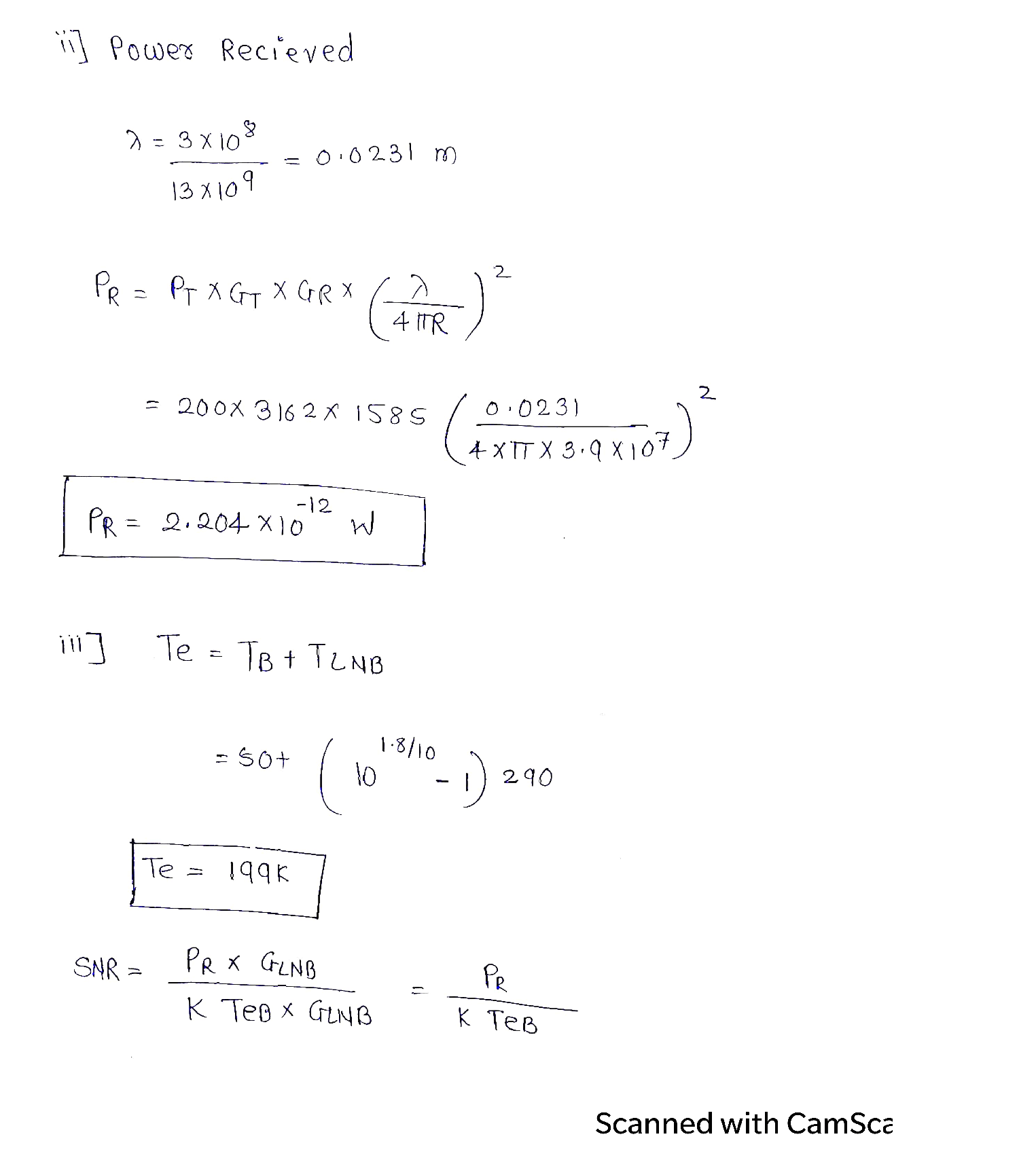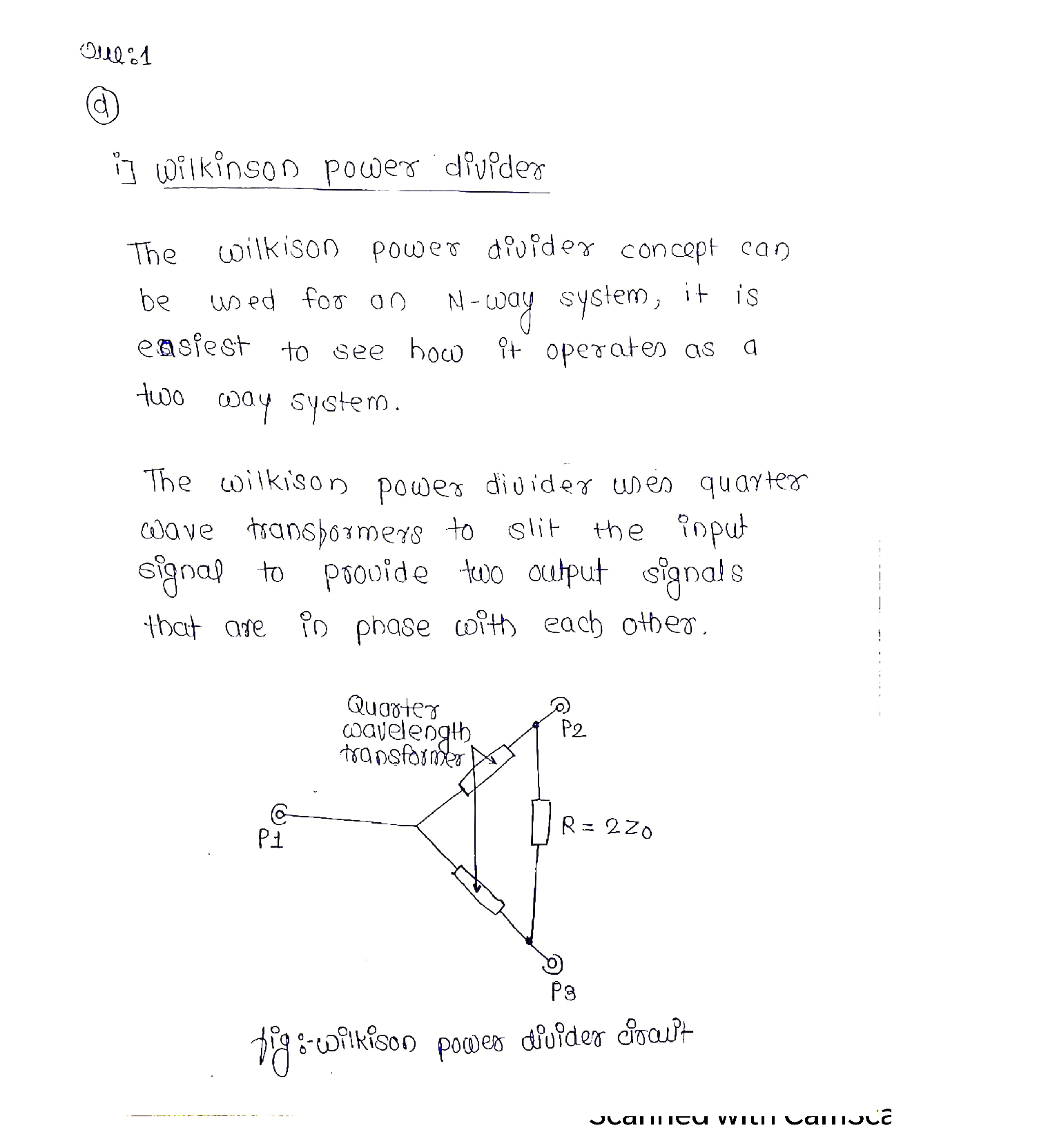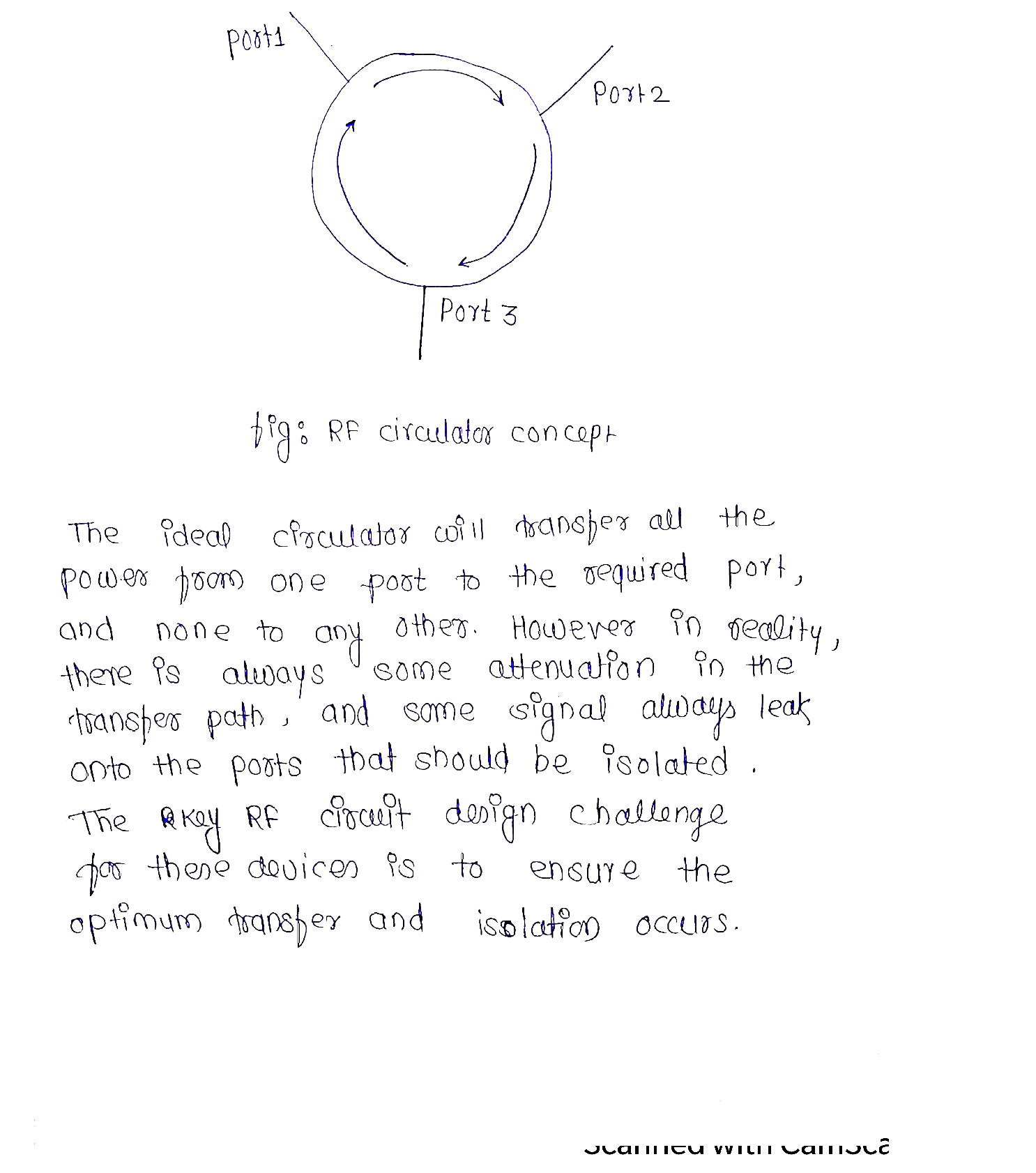### Question 41783Antenna Theory

). Design a 10 × 8 (10 in the x-direction and 8 in the y) element uniform planar array so that the main maximum is oriented along thetag = 10°, phi o = 90°. For a spacing of d, = d, = 1/8between the elements, find the
(a) progressive phase shift between the elements in the x and y directions
(b) directivity of the array
(c) half-power beamwidths (in two perpendicular planes) of the array.

### Question 41782Antenna Theory

Design a five-element binomial array with elements placed along the z-axis.
(a) Derive the excitation coefficients.
(b) Write a simplified expression for the array factor.
(c) For a spacing of d = 31/4, determine all the angles theta (in degrees) where the array factor possesses nulls.
(d) For a spacing of d = 31/4, determine all the angles theta (in degrees) where the array factor possesses main maxima.

### Question 41781Antenna Theory

An array of 10 isotropic elements are placed along the z-axis a distance d apart. Assum-ing uniform distribution, find the progressive phase (in degrees), half-power beam width (in degrees), first-null beam width (in degrees), first side lobe level maximum beam width (indegrees), relative side lobe level maximum (in dB), and directivity (in dB) (using equations and the computer program Directivity of Chapter 2, and compare) for
(b) ordinary end-fire
(c) Hansen-Woodyard end-fire

### Question 41780Antenna Theory

It is desired to design a uniform ordinary end-fire array of 6 elements with a maximum toward theta o = 0° and 0, = 180°, simultaneously. Determine the
(b) excitation progressive phase shift (in degrees) that should be used
(c) approximate directivity of the array (dimensionless and in dB)
(d) relative value (in dB) of the magnitude of the array factor toward broadside (0, = 90°)compared to that toward the maximum (theta, = 0° or 180°).
(a) smallest separation between the elements (in 1).

### Question 41779Antenna Theory

Design a uniform broadside linear array of N elements placed along the z-axis with a uniformspacing d = /10 between the elements. Determine the closest integer number of elementsso that in the elevation plane the
(a) Half-power beam width of the array factor is approximately 60°.
(b) First-null beam width of the array factor is 60°.

### Question 41778Antenna Theory

(a) Find the array factor.(b) Find all the nulls.
A three-element array of isotropic sources has the phase and magnitude relationships shown.The spacing between the elements is d = lambda/2.(a) Find the array factor.(b) Find all the nulls.

### Question 40893Antenna Theory

There is a requirement to design a digital broadcast transmission system that uses QuadratureAmplitude Modulation (QAM) and Orthogonal Frequency Division Multiplexing (OFDM).
a) Explain with the aid of diagrams how QAM encodes and decodes data and explain the difference between data rate and symbol rate.(8 marks)
b) Explain with aid of diagrams why Orthogonal Frequency Division Multiplexing (OFDM)would be a suitable modulation scheme for a digital broadcast transmission system and why the symbol duration is an important design consideration?(8 marks)
c) If the transmission system was capable of transmitting 64kbps with a symbol rate of 4ms.(Please show and explain all calculations for the following):
b. Explain and justify the QAM encoding level required to achieve the above.
Determine and justify the number of OFDM carriers.

### Question 40892Antenna Theory

Electro Magnetic Interference (EMI) is normally considered as being either conductive or radiative. For each type of interference describe ways of reducing their effects.
A carrier of frequency 800kHz is being Amplitude Modulated (AM) by a modulating signal that is a sine wave at a constant frequency of 8kHz and constant amplitude. The modulation index is less than 100%. Determine the frequency of the upper sideband and lower sideband and total bandwidth of the resultant Amplitude Modulated(3 marks)waveform.
If the carrier in part (b) has a peak-to-peak voltage of 10V and the modulating signal has a peak-to-peak voltage of 5V, determine the modulation index, the maximum and minimum voltages of the resultant Amplitude Modulated waveform and the peak amplitude of the upper and lower side bands. Hence, determine the modulating signal voltage that would result in 100% modulation.(7 marks)
Briefly describe the process by which the modulating signal would be extracted from the Amplitude Modulated waveform at a receiver. Using the signal described in part(b) and if the demodulator comprises a simple diode detector, determine a value for the demodulator's time constant and justify your choice. Hence, determine the value of capacitance required if the resistance = 2k.(9 marks)

### Question 40891Antenna Theory

If the Signal to Noise Ratio (SNR) of a wireless link is 20DB and the Radio Frequency(RF) bandwidth is 20kHz, using Shannon's capacity formula, determine the maximum theoretical data rate that can be transmitted.(5 marks)
b)With the aid of block diagrams explain the principle operation of (DSSS) Direct Sequence Spread Spectrum and (FHSS) Frequency Hopping Spread Spectrum transmission techniques.(12 marks)
With the aid of a diagram and a brief explanation, what is the purpose of the autocorrelation function, and how does it work in a DSSS WLAN.(8 marks)

### Question 40890Antenna Theory

With the aid of a diagram, show how a transmission line can be represented as anelectric circuit; and list the electrical properties of the transmission line with appropriateunits of measurement.(4 marks)
When considering a pulse moving along a transmission line; with the aid of a diagram,explain the difference between a lumped and distributed system with reference to thetwo-way delay time 2·t and the rise time of the pulse.(6 marks)
With the aid of diagrams, explain what happens when the load of a terminatedtransmission line:
If a correctly terminated line has a characteristic impedance of 50 ohms and a measuredvelocity of propagation of 2.2 x 108 m/s with an attenuation of 6dB/km. If a faultdevelops, and the reflected pulses are returned non-inverted after 10us with amagnitude 0.025 of that of the transmitted pulse. Calculate the resistance and position(9 marks)of the fault
(ii) Decreases in resistance.
(i) Increases in resistance.

### Submit query

Getting answers to your urgent problems is simple. Submit your query in the given box and get answers Instantly.

### Submit a new QuerySuccess

Assignment is successfully created